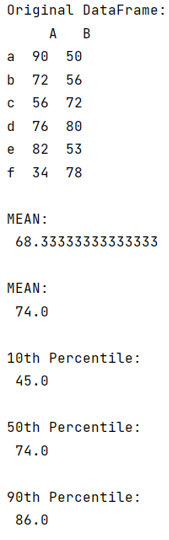# Pandas: Find percentile stats of a given column

Given a Pandas DataFrame, we have to find percentile stats of a given column.
Submitted by Pranit Sharma, on July 07, 2022

Pandas is a special tool that allows us to perform complex manipulations of data effectively and efficiently. Inside pandas, we mostly deal with a dataset in the form of DataFrame. DataFrames are 2-dimensional data structures in pandas. DataFrames consist of rows, columns, and data.

Pandas allows us to perform almost every kind of mathematical operations including statistical operations like mean, median, and mode.

To find the percentile stats of a given column, we will use methods like mean(), median(), and mode().

1) pandas.DataFrame.mean() Method

This method returns the mean of the values over the requested axis.

```DataFrame.mean(
axis=NoDefault.no_default,
skipna=True,
level=None,
numeric_only=None,
**kwargs
)
```

2) pandas.DataFrame.median() Method

This method returns the median of the values over the requested axis.

```DataFrame.median(
axis=NoDefault.no_default,
skipna=True,
level=None,
numeric_only=None,
**kwargs
)
```

3) pandas.DataFrame.mode() Method

This method returns the mode(s) of each element along the selected axis.

```DataFrame.mode(
axis=0,
numeric_only=False,
dropna=True
)
```

To work with pandas, we need to import pandas package first, below is the syntax:

```import pandas as pd
```

Let us understand with the help of an example,

## Python code to find percentile stats of a given column

```# Importing pandas package
import pandas as pd

# Creating a dictionary
d = {
'A':[90,72,56,76,82,34],
'B':[50,56,72,80,53,78]
}

# Creating a DataFrame
df = pd.DataFrame(d,index=['a','b','c','d','e','f'])

# Display original DataFrame
print("Original DataFrame:\n",df,"\n")

# Get multiple statistical result

print("MEAN:\n",df['A'].mean(),"\n")

print("MEAN:\n",df['A'].median(),"\n")

# Calculating percentile using quantile

print("10th Percentile:\n",df['A'].quantile(0.1),"\n")

print("50th Percentile:\n",df['A'].quantile(0.5),"\n")

print("90th Percentile:\n",df['A'].quantile(0.9),"\n")
```

Output:What's New (MCQs)

Top Interview Coding Problems/Challenges!

IncludeHelp's Blogs

Languages: » C » C++ » C++ STL » Java » Data Structure » C#.Net » Android » Kotlin » SQL
Web Technologies: » PHP » Python » JavaScript » CSS » Ajax » Node.js » Web programming/HTML
Solved programs: » C » C++ » DS » Java » C#
Aptitude que. & ans.: » C » C++ » Java » DBMS
Interview que. & ans.: » C » Embedded C » Java » SEO » HR
CS Subjects: » CS Basics » O.S. » Networks » DBMS » Embedded Systems » Cloud Computing
» Machine learning » CS Organizations » Linux » DOS
More: » Articles » Puzzles » News/Updates